Illustrated probability calculations from distributions

pdist(
dist = "norm",
q,
plot = TRUE,
verbose = FALSE,
invisible = FALSE,
digits = 3L,
xlim,
ylim,
resolution = 500L,
return = c("values", "plot"),
...,
refinements = list()
)

xpgamma(
q,
shape,
rate = 1,
scale = 1/rate,
lower.tail = TRUE,
log.p = FALSE,
...
)

xpt(q, df, ncp, lower.tail = TRUE, log.p = FALSE, ...)

xpchisq(q, df, ncp = 0, lower.tail = TRUE, log.p = FALSE, ...)

xpf(q, df1, df2, lower.tail = TRUE, log.p = FALSE, ...)

xpbinom(q, size, prob, lower.tail = TRUE, log.p = FALSE, ...)

xppois(q, lambda, lower.tail = TRUE, log.p = FALSE, ...)

xpgeom(q, prob, lower.tail = TRUE, log.p = FALSE, ...)

xpnbinom(q, size, prob, mu, lower.tail = TRUE, log.p = FALSE, ...)

xpbeta(q, shape1, shape2, ncp = 0, lower.tail = TRUE, log.p = FALSE, ...)

## Arguments

dist

a character description of a distribution, for example "norm", "t", or "chisq"

q

a vector of quantiles

plot

a logical indicating whether a plot should be created

verbose

a logical

invisible

a logical

digits

the number of digits desired

xlim

x limits

ylim

y limits

resolution

Number of points used for detecting discreteness and generating plots. The default value of 5000 should work well except for discrete distributions that have many distinct values, especially if these values are not evenly spaced.

return

If "plot", return a plot. If "values", return a vector of numerical values.

...

Additional arguments, typically for fine tuning the plot.

refinements

A list of refinements to the plot. See ggformula::gf_refine().

shape

shape and scale parameters. Must be positive, scale strictly.

rate

an alternative way to specify the scale.

scale

shape and scale parameters. Must be positive, scale strictly.

lower.tail

logical; if TRUE (default), probabilities are $$P[X \le x]$$, otherwise, $$P[X > x]$$.

log.p

logical; if TRUE, probabilities/densities $$p$$ are returned as $$log(p)$$.

df

degrees of freedom ($$> 0$$, maybe non-integer). df = Inf is allowed.

ncp

non-centrality parameter $$\delta$$; currently except for rt(), only for abs(ncp) <= 37.62. If omitted, use the central t distribution.

df1

degrees of freedom. Inf is allowed.

df2

degrees of freedom. Inf is allowed.

size

number of trials (zero or more).

prob

probability of success on each trial.

lambda

vector of (non-negative) means.

mu

alternative parametrization via mean: see ‘Details’.

shape1

non-negative parameters of the Beta distribution.

shape2

non-negative parameters of the Beta distribution.

## Value

A vector of probabilities; a plot is printed as a side effect.

## Details

The most general function is pdist which can work with any distribution for which a p-function exists. As a convenience, wrappers are provided for several common distributions.

qdist(), xpnorm(), xqnorm().

## Examples

pdist("norm", -2:2)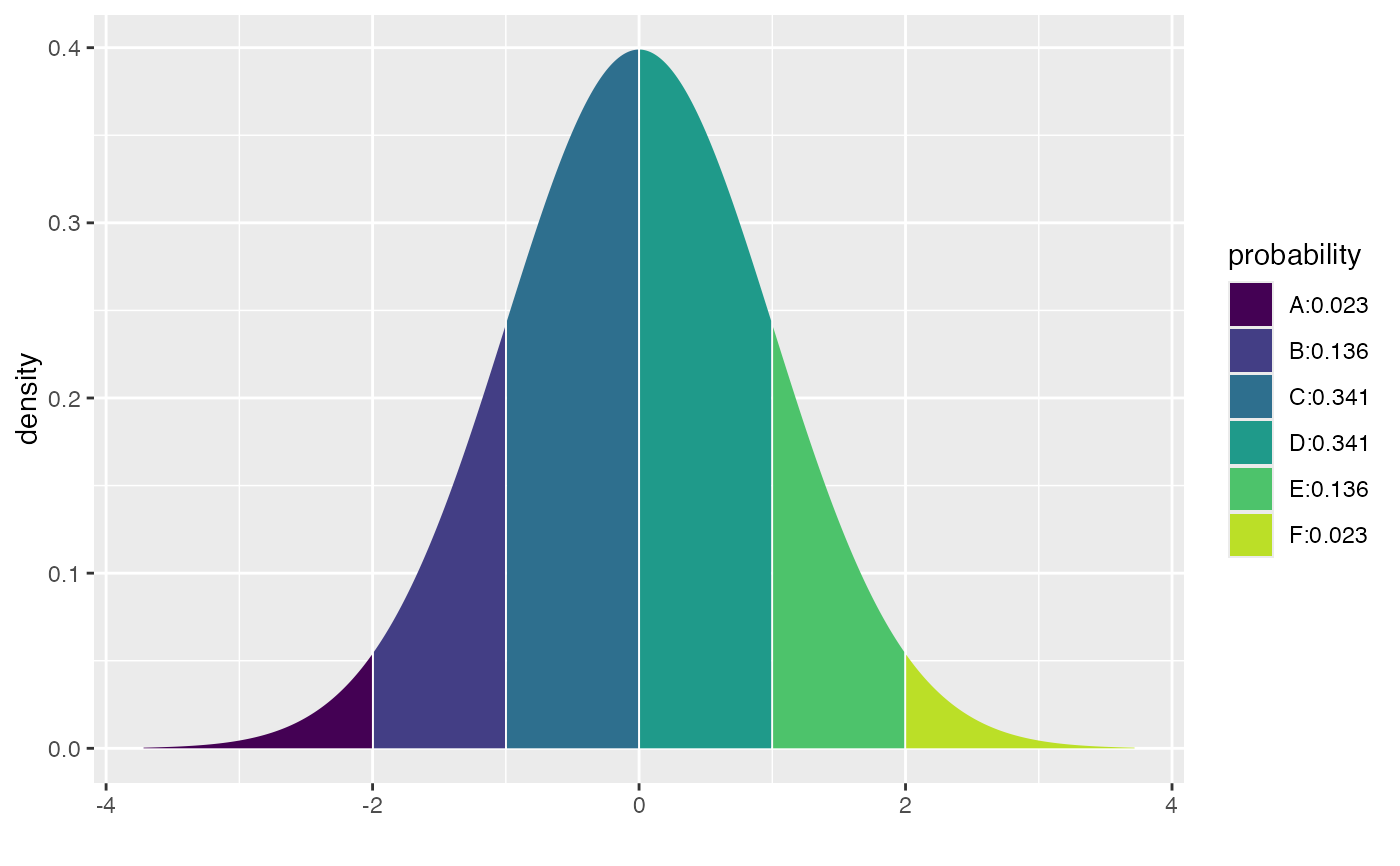#>  0.02275013 0.15865525 0.50000000 0.84134475 0.97724987
pdist("norm", seq(80,120, by = 10), mean = 100, sd = 10)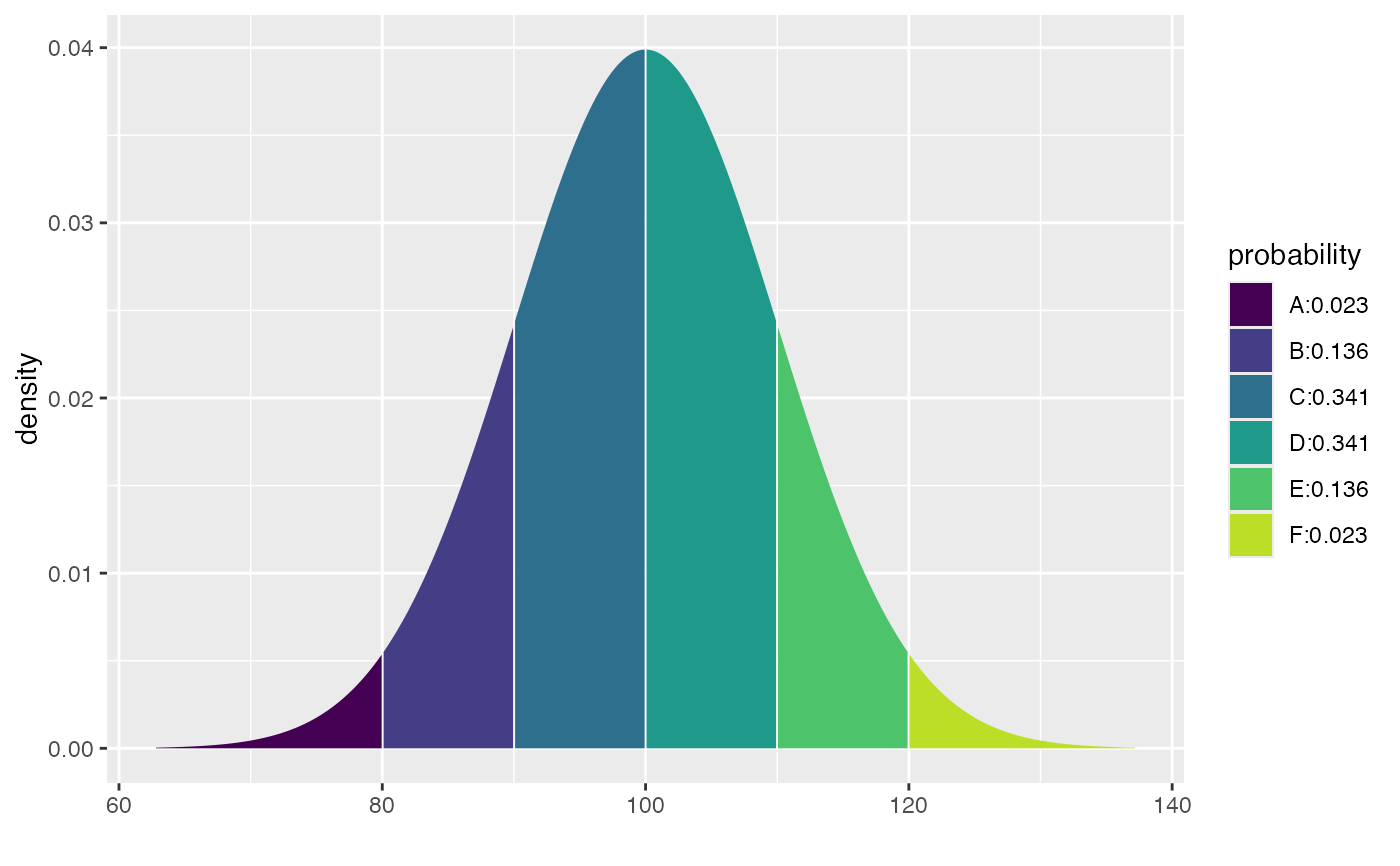#>  0.02275013 0.15865525 0.50000000 0.84134475 0.97724987
pdist("chisq", 2:4, df = 3)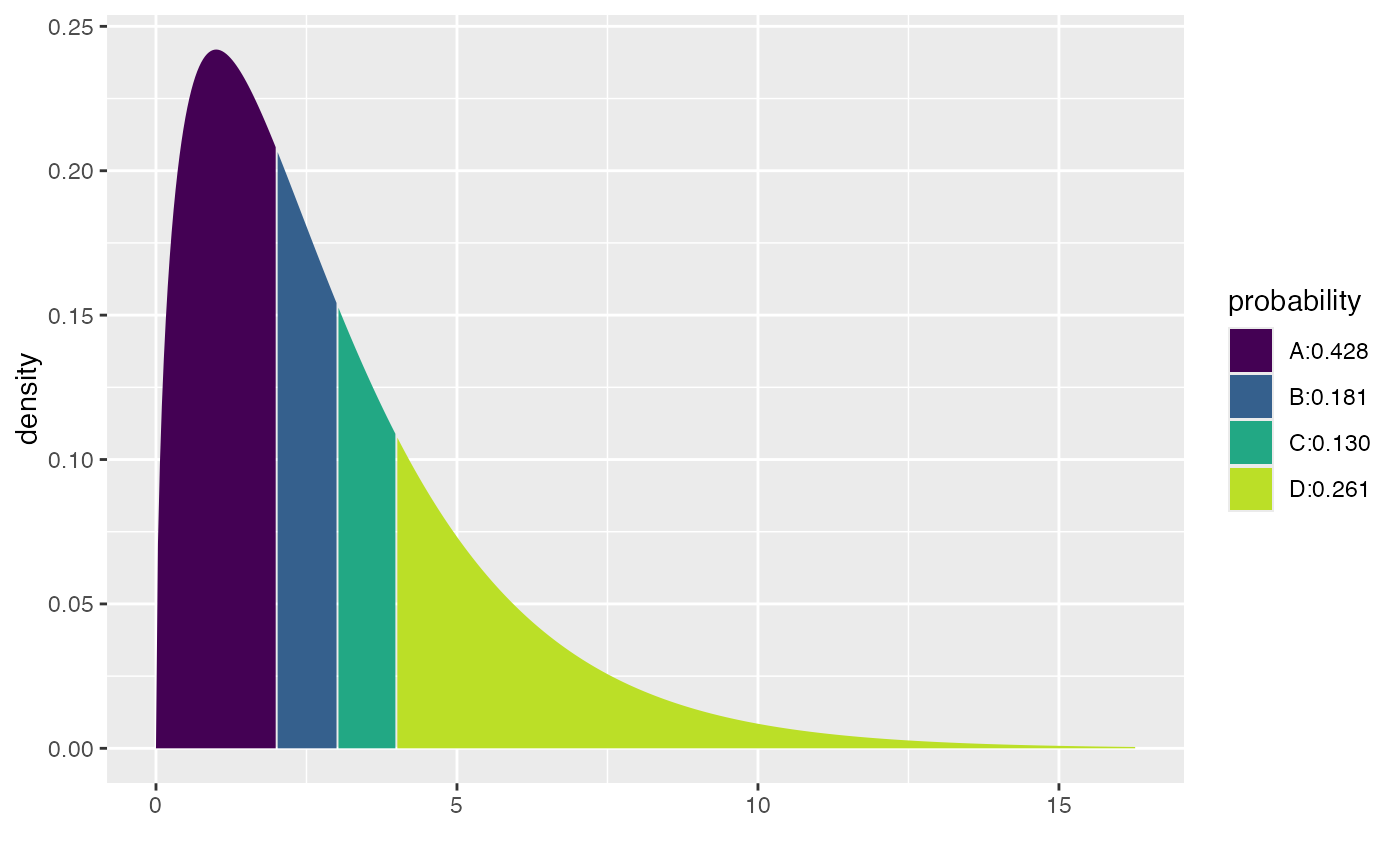#>  0.4275933 0.6083748 0.7385359
pdist("f", 1, df1 = 2, df2 = 10)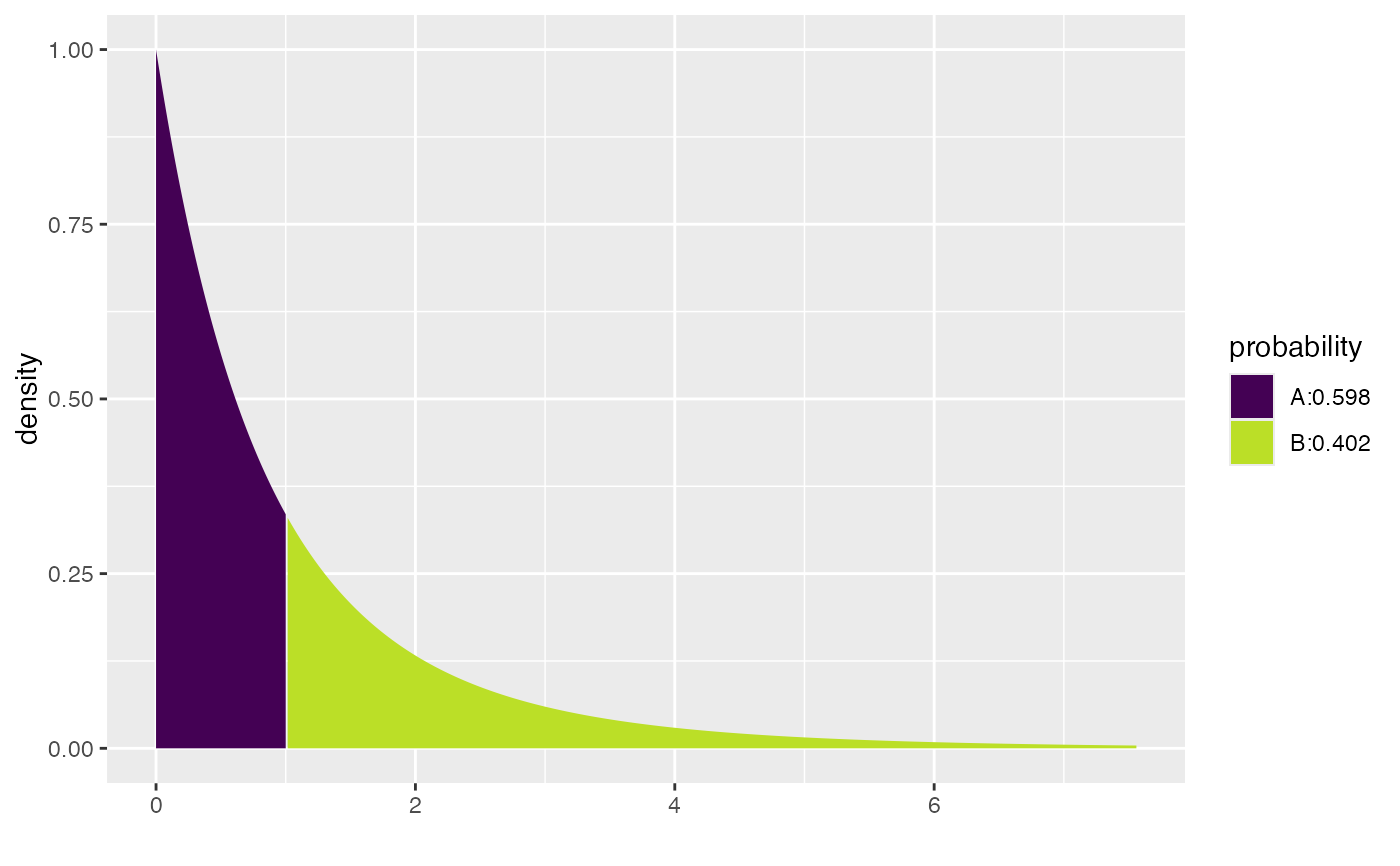#>  0.5981224
pdist("gamma", 2, shape = 3, rate = 4)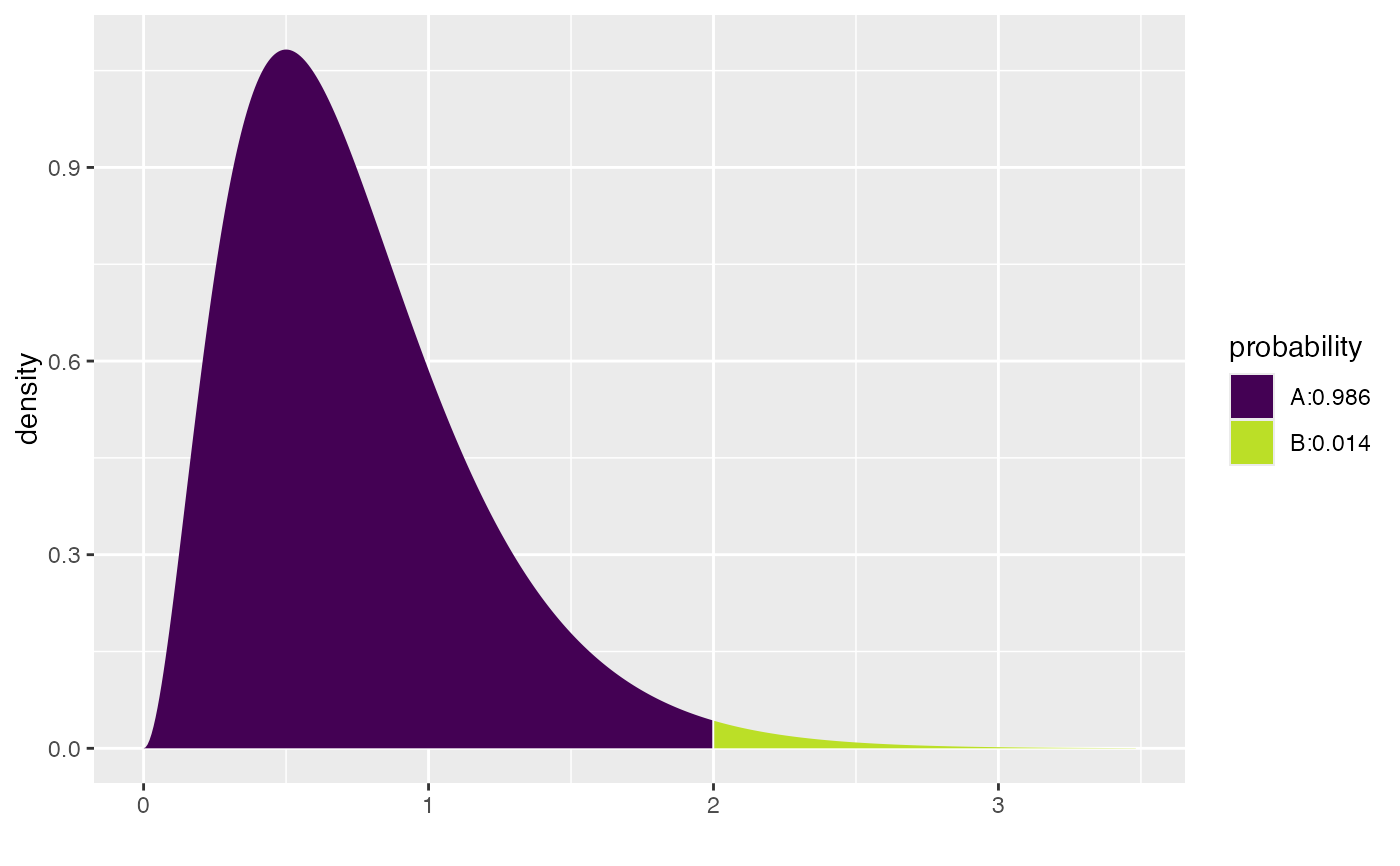#>  0.986246